Question

Three six-faced fair dice are rolled. What is the probability that exactly two of the dice have the face value of 6?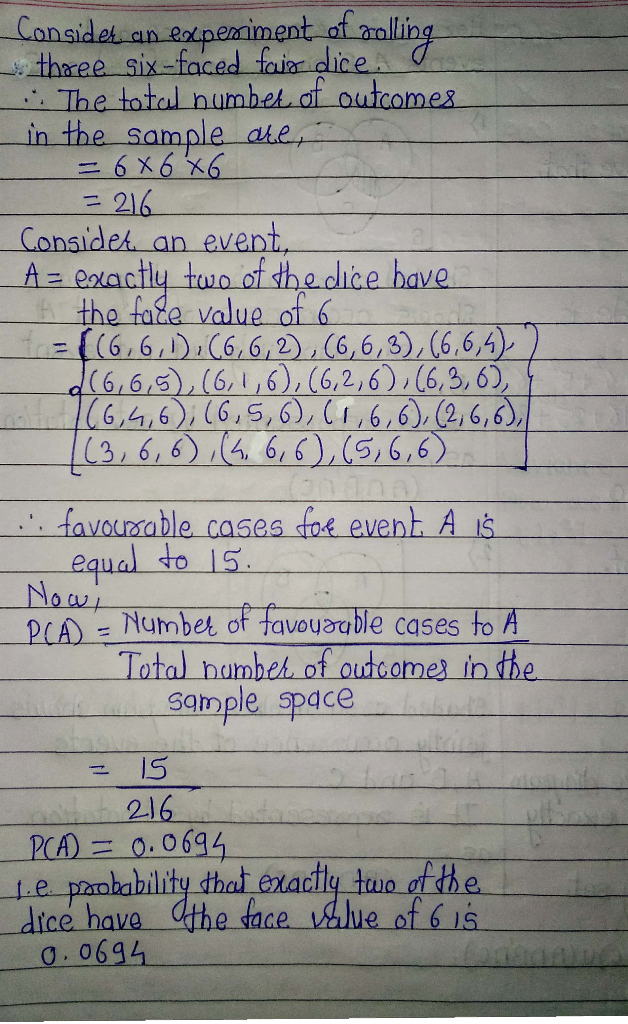If you are satisfied with the answer then press like button please please

#### Earn Coins

Coins can be redeemed for fabulous gifts.

Similar Homework Help Questions
• ### Two fair six-sided dice are rolled. What is the probability that one die shows exactly three...

Two fair six-sided dice are rolled. What is the probability that one die shows exactly three more than the other die (for example, rolling a 1 and 4, or rolling a 6 and a 3)

• ### Three fair six-sided dice are rolled. a) What is the probability of seeing {1, 3, 6}?...

Three fair six-sided dice are rolled. a) What is the probability of seeing {1, 3, 6}? b) What is the probability of seeing {1, 4, 4}? c) What is the probability of seeing {2, 2, 2} ? d) What is the probability of seeing at least one 6? e) What is the probability that the sum of all three dice is 16? f) What is the probability of seeing exactly two even numbers?

• ### Two fair (6-sided) dice are rolled. If you are told that the two dice end up...

Two fair (6-sided) dice are rolled. If you are told that the two dice end up with two different face values, what is the probability that one die has a face value of 6?

• ### Problem 1 (10 points). If two fair dice are rolled 10 times, what is the probability...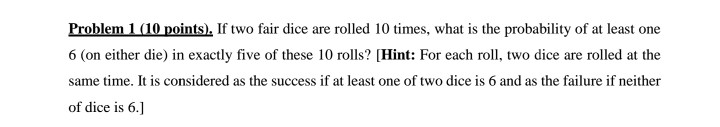Problem 1 (10 points). If two fair dice are rolled 10 times, what is the probability of at least one 6 (on either die) in exactly five of these 10 rolls? (Hint: For each roll, two dice are rolled at the same time. It is considered as the success if at least one of two dice is 6 and as the failure if neither of dice is 6.]

• ### 4. Three fair dice have different colors: red, blue, and yellow. These three dice are rolled...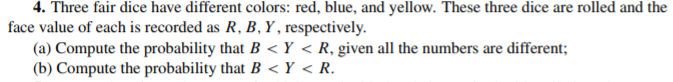4. Three fair dice have different colors: red, blue, and yellow. These three dice are rolled and the face value of each is recorded as R, B, Y, respectively. (a) Compute the probability that B< Y < R, given all the numbers are different; (b) Compute the probability that B< Y< R.

• ### A six-sided dice is rolled twice. Find the probability that the larger of the two rolls...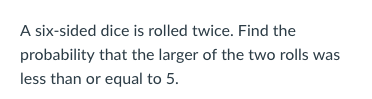A six-sided dice is rolled twice. Find the probability that the larger of the two rolls was less than or equal to5 A fair coin is flipped 3 times. Find the probability that exactly 1 of the flips will turn up as heads.

• ### Conditional Probability Two fair dice are rolled: (a) Express the sample space S in set builder...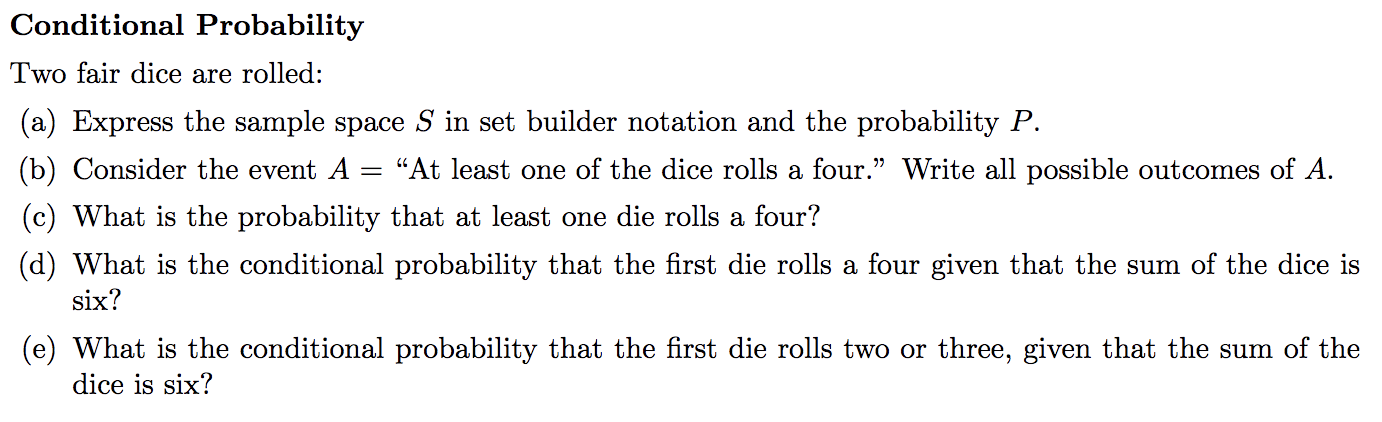Conditional Probability Two fair dice are rolled: (a) Express the sample space S in set builder notation and the probability P "At least one of the dice rolls a four." Write all possible outcomes of A (b) Consider the event A (c) What is the probability that at least one die rolls a four? (d) What is the conditional probability that the first die rolls a four given that the sum of the dice is six? (e) What is the...

• ### if you have two fair dice that are rolled, what is the probability of a sum 6 given that the roll is a 'double'

if you have two fair dice that are rolled, what is the probability of a sum 6 given that the roll is a 'double'?

• ### 1.) Suppose you roll two fair six-sided dice. What is the probabilty that I rolled a...

1.) Suppose you roll two fair six-sided dice. What is the probabilty that I rolled a total of 5? 2.) Suppose you roll two fair six-sided die and I announce that the sun of the two die is 6 or less. What is the probabilty that you rolled a total of 5?

• ### If two fair dice are rolled, find the probability that the sum of the dice is...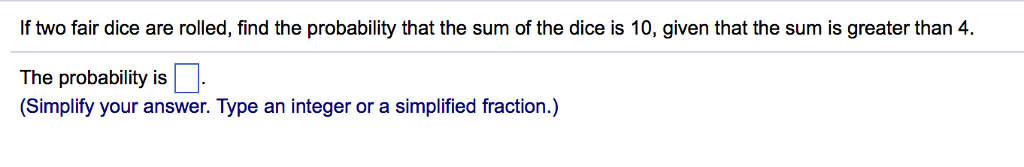If two fair dice are rolled, find the probability that the sum of the dice is 10, given that the sum is greater than 4. The probability is (Simplify your answer. Type an integer or a simplified fraction.)